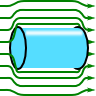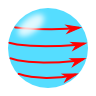# Magnetic Fields in Matter

In dielectric media, dipoles get aligned by an external electric field - a polarization density $$\mathbf{P}\left(\mathbf{r}\right)=\varepsilon_{0}\chi\left(\mathbf{r}\right)\mathbf{E}\left(\mathbf{r}\right)$$ builds up. In magnetic media, the same happens with the magnetic field and magnetic dipoles. Here, however, the effect is called magnetization $$\mathbf{M}\left(\mathbf{r}\right)=\chi_{m}\left(\mathbf{r}\right)\mathbf{H}\left(\mathbf{r}\right)$$ with the "magnetic suszeptibility" $$\chi_{m}\left(\mathbf{r}\right)$$. Then, the magnetic induction is given by $\begin{eqnarray*}\mathbf{B}\left(\mathbf{r}\right)&=&\mu_{0}\left(\mathbf{H}\left(\mathbf{r}\right)+\mathbf{M}\left(\mathbf{r}\right)\right)\\&=&\mu_{0}\left(\mathbf{H}\left(\mathbf{r}\right)+\chi_{m}\left(\mathbf{r}\right)\mathbf{H}\left(\mathbf{r}\right)\right)\\&\equiv&\mu_{0}\color{red}{\mu\left(\mathbf{r}\right)}\mathbf{H}\left(\mathbf{r}\right)\end{eqnarray*}$with the introduced relative permeability $$\mu\left(\mathbf{r}\right)$$. If $$\mu\left(\mathbf{r}\right)\neq1$$ and so $$\chi_{m}\left(\mathbf{r}\right)$$ does not vanish, we speak of a magnetic medium. In the given problems we will use Maxwell's equations in the magnetostatic approximation to calculate the magnetic field(s) with present magnetic media.

## Superconductors and Their Magnetostatic FieldsThe Proca-formulation of electrodynamics allows to account for a hypothetical massive photon. This formulation lead to astonishing experiments but is formally equivalent to the London theory of superconductivity. Learn in this problem how a magnetic field is expelled from a superconductor by "massive photons".

## A Magnetic Sphere with Surface CurrentUsing the magnetostatic potential can be extremely useful to calculate magnetostatic problems. However, it can only be defined if no currents are present. In this problem you will discover how we can still use the potential in situations of strongly confined surface currents.